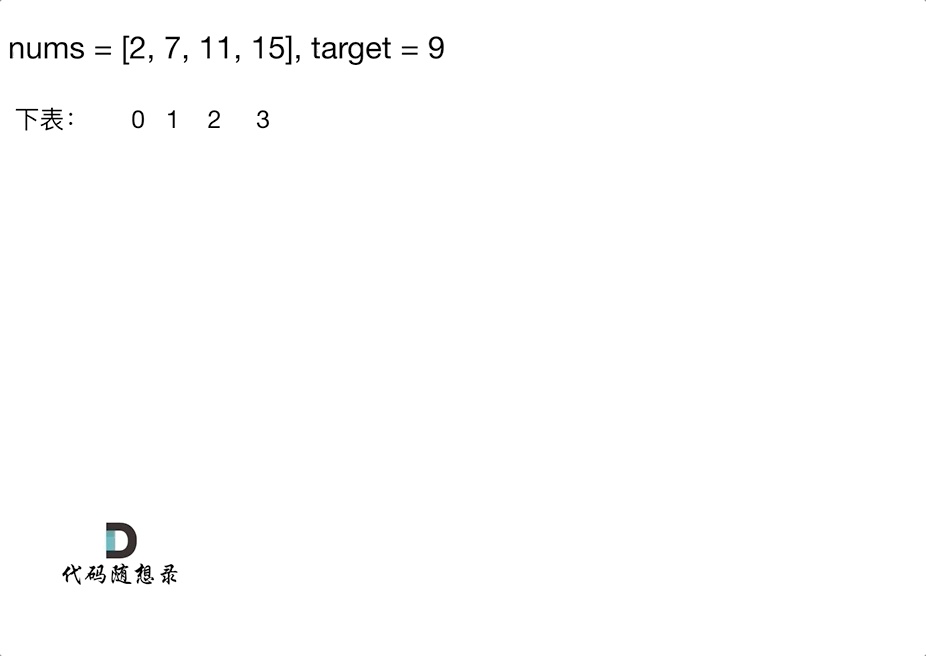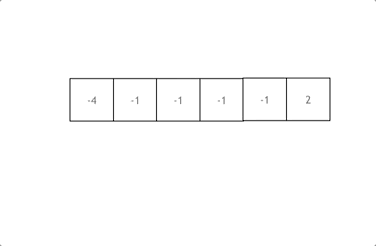# Hash-题解-方法

2021-09-10 22:40 113 查看

# 有效的字母异位词

```class Solution {
public boolean isAnagram(String s, String t) {

int[] record = new int;
for (char c : s.toCharArray()) {
record[c - 'a'] += 1;
}
for (char c : t.toCharArray()) {
record[c - 'a'] -= 1;
}
for (int i : record) {
if (i != 0) {
return false;
}
}
return true;
}
}```

# 两个数组的交集

```import java.util.HashSet;
import java.util.Set;

class Solution {
public int[] intersection(int[] nums1, int[] nums2) {
if (nums1 == null || nums1.length == 0 || nums2 == null || nums2.length == 0) {
return new int;
}
Set<Integer> set1 = new HashSet<>();
Set<Integer> resSet = new HashSet<>();
//遍历数组1
for (int i : nums1) {
}
//遍历数组2的过程中判断哈希表中是否存在该元素
for (int i : nums2) {
if (set1.contains(i)) {
}
}
int[] resArr = new int[resSet.size()];
int index = 0;
//将结果几何转为数组
for (int i : resSet) {
resArr[index++] = i;
}
return resArr;
}
}```

# 快乐数

「快乐数」定义为：对于一个正整数，每一次将该数替换为它每个位置上的数字的平方和，然后重复这个过程直到这个数变为 1，也可能是 无限循环 但始终变不到 1。如果 可以变为 1，那么这个数就是快乐数。

```class Solution {
public boolean isHappy(int n) {
Set<Integer> record = new HashSet<>();
while (n != 1 && !record.contains(n)) {
n = getNextNumber(n);
}
return n == 1;
}

private int getNextNumber(int n) {
int res = 0;
while (n > 0) {
int temp = n % 10;
res += temp * temp;
n = n / 10;
}
return res;
}
}```

# 两数之和

• 数组的大小是受限制的，而且如果元素很少，而哈希值太大会造成内存空间的浪费。
• set是一个集合，里面放的元素只能是一个key，而两数之和这道题目，不仅要判断y是否存在而且还要记录y的下表位置，因为要返回x 和 y的下表。所以set 也不能用。# 四数相加II

1. (0, 0, 0, 1) -> A + B + C + D = 1 + (-2) + (-1) + 2 = 0
2. (1, 1, 0, 0) -> A + B + C + D = 2 + (-1) + (-1) + 0 = 0

1. 首先定义 一个map，key放a和b两数之和，value 放a和b两数之和出现的次数。
2. 遍历大A和大B数组，统计两个数组元素之和，和出现的次数，放到map中。
3. 定义int变量count，用来统计a+b+c+d = 0 出现的次数。
4. 在遍历大C和大D数组，找到如果 0-(c+d) 在map中出现过的话，就用count把map中key对应的value也就是出现次数统计出来。
5. 最后返回统计值 count 就可以了
```class Solution {
public int fourSumCount(int[] nums1, int[] nums2, int[] nums3, int[] nums4) {
Map<Integer, Integer> map = new HashMap<>();
int temp;
int res = 0;
//统计两个数组中的元素之和，同时统计出现的次数，放入map
for (int i : nums1) {
for (int j : nums2) {
temp = i + j;
if (map.containsKey(temp)) {
map.put(temp, map.get(temp) + 1);
} else {
map.put(temp, 1);
}
}
}
//统计剩余的两个元素的和，在map中找是否存在相加为0的情况，同时记录次数
for (int i : nums3) {
for (int j : nums4) {
temp = i + j;
if (map.containsKey(0 - temp)) {
res += map.get(0 - temp);
}
}
}
return res;
}
}```

# 赎金信

(题目说明：为了不暴露赎金信字迹，要从杂志上搜索各个需要的字母，组成单词来表达意思。杂志字符串中的每个字符只能在赎金信字符串中使用一次。)

canConstruct("a", "b") -> false canConstruct("aa", "ab") -> false canConstruct("aa", "aab") -> true

```class Solution {
public boolean canConstruct(String ransomNote, String magazine) {
//记录杂志字符串出现的次数
int[] arr = new int;
int temp;
for (int i = 0; i < magazine.length(); i++) {
temp = magazine.charAt(i) - 'a';
arr[temp]++;
}
for (int i = 0; i < ransomNote.length(); i++) {
temp = ransomNote.charAt(i) - 'a';
//对于金信中的每一个字符都在数组中查找
//找到相应位减一，否则找不到返回false
if (arr[temp] > 0) {
arr[temp]--;
} else {
return false;
}
}
return true;
}
}```

# 三数之和• 拿这个nums数组来举例，首先将数组排序，然后有一层for循环，i从下标0的地方开始，同时定一个下标left 定义在i+1的位置上，定义下标right 在数组结尾的位置上。

• 依然还是在数组中找到 abc 使得a + b +c =0，我们这里相当于 a = nums[i] b = nums[left] c = nums[right]。

• 接下来如何移动left 和right呢， 如果nums[i] + nums[left] + nums[right] > 0 就说明 此时三数之和大了，因为数组是排序后了，所以right下标就应该向左移动，这样才能让三数之和小一些。

• 如果 nums[i] + nums[left] + nums[right] < 0 说明 此时 三数之和小了，left 就向右移动，才能让三数之和大一些，直到left与right相遇为止。

```class Solution {
public List<List<Integer>> threeSum(int[] nums) {
List<List<Integer>> result = new ArrayList<>();
Arrays.sort(nums);

for (int i = 0; i < nums.length; i++) {
if (nums[i] > 0) {
return result;
}

if (i > 0 && nums[i] == nums[i - 1]) {
continue;
}

int left = i + 1;
int right = nums.length - 1;
while (right > left) {
int sum = nums[i] + nums[left] + nums[right];
if (sum > 0) {
right--;
} else if (sum < 0) {
left++;
} else {

while (right > left && nums[right] == nums[right - 1]) right--;
while (right > left && nums[left] == nums[left + 1]) left++;

right--;
left++;
}
}
}
return result;
}
}```